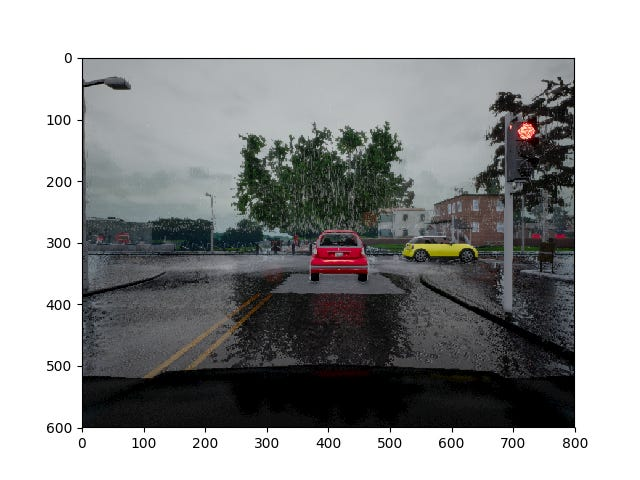# FCN Based Semantic Segmentation Using Bayesian Optimization for Hyperparameter Tuning

INTRODUCTION
Image segmentation is an important subfield of computer vision. With the advent of Deep Learning, like most areas of computer vision, image segmentation has been significantly impacted. This article focuses on applying deep learning based image segmentation to autonomous vehicle applications. In particular, this article discusses my implementation for the Lyft-Udacity perception challenge that took place in June of this year.

The objective of the challenge was to develop a semantic segmentation algorithm that can recognize in real-time vehicles and drivable areas of the road, in a simulated urban environment under different weather conditions. In particular, given an image from the front facing camera on an autonomous vehicle, for every pixel, the goal is to identify it into a vehicle, road, or something else category. Per the challenge requirement, the figure-of-merit used was a weighted F-score that placed more weightage on the vehicle precision — refer to the GitHub code for details.

The architecture used is based upon Long et al’s FCN-8 architecture . In addition to the core FCN-8 architecture, I used Bayesian optimization for find the best hyperparameter — see the section on Bayesian optimization for details. In this article, I’ll focus on a high level overview of the architecture and methods used for training the network. Interested readers can find more details on my GitHub.

DATA AUGMENTATION
The dataset provided for this competition is from the Carla simulator. The ground-truth dataset is pre-processed to only include three classes: vehicle, road, and everything else. The dataset is divided into training, validation, and test set. To help the model generalize better (i.e. improve F-score on the validation and test sets), data augmentation is done. In particular, images are randomly (horizontally) flipped, rotated by +/-20 degrees, shifted/translated by +/-10% of image size in both dimensions, and add random noise to the pixel values.

Data augmentation is randomly performed 50% of the time during training. This allows the model to learn from a wider distribution of images. Note that data pre-processing (i.e. input normalization) is done as part of the input layer of the network inside TensorFlow. Figure 1 shows the augmented input images and their corresponding (augmented) ground truth images. For the ground truth images, yellow corresponds to vehicle, turquoise to road, and purple to the everything else category.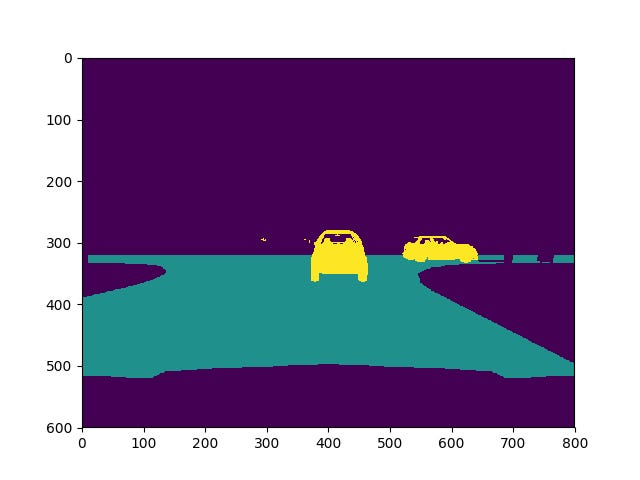Left: “Figure 1 (a.1): Original (Input Image)” & Right: “Figure 1 (a.2): Original (Ground Truth)”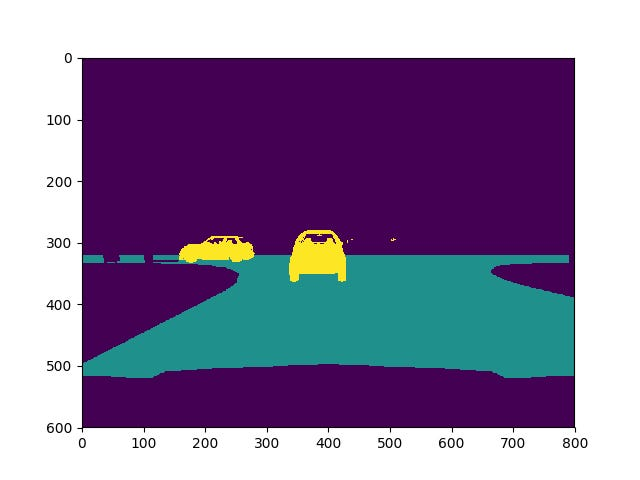Left: ”Figure 1 (b.1): Horizontal Flip (Input Image)” & Right: “Figure 1 (b.2): Horizontal Flip (Ground Truth)”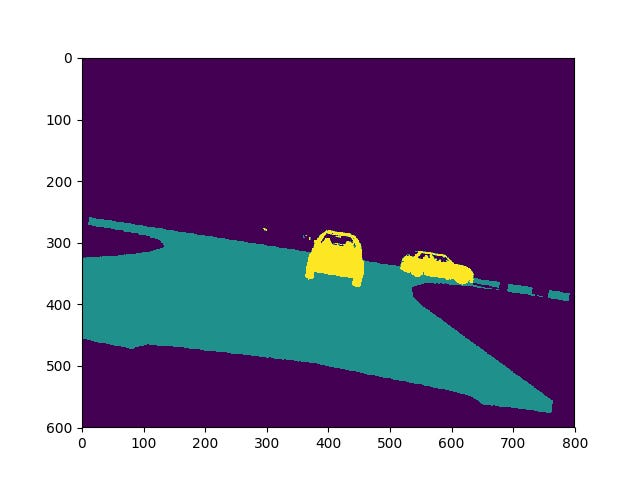Left: “Figure 1 (c.1): Rotate (Input Image)” & Right: “Figure 1 (c.2): Rotate (Ground Truth)”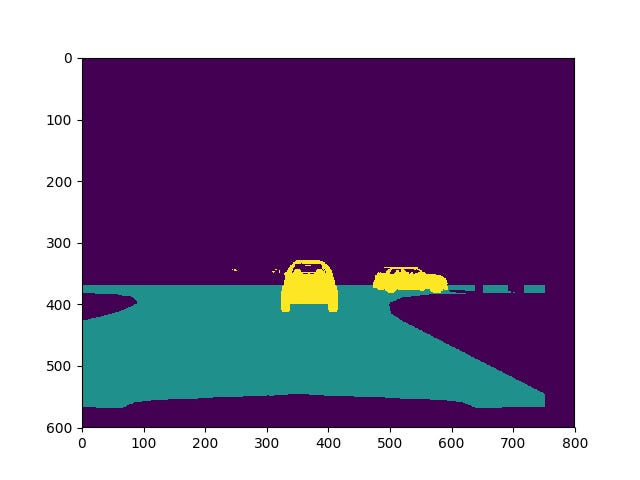Left: “Figure 1 (d.1): Translate (Input Image)” & Right: “Figure 1 (d.2): Translate (Ground Truth)”Left: “Figure 1 (e.1): Additive Noise (Input Image)” & Right: “Figure 1 (e.2): Additive Noise (Ground Truth)”

FCN-8 ARCHITECTURE
As stated earlier, the architecture used for image segmentaiton is based upon Long et al’s FCN-8 architecture . It basically takes a VGG16 network and replaces the fully-connected layers with fully-convolutional layers. The output of the fully convolutional layer is then upsampled back to the input image’s dimension using a learnable kernel (i.e. transposed convolution). As illustrated in Figure 2, to help improve the accuracy in detecting smaller scale components, there are a couple skip layers which upsamples the output from earlier layers in the VGG16 network and combines/fuses all of them together (using matrix addition) at different places.

The output layer of the vggnet (layer 7) is converted into a fully convolutional layer with a 1×1 convolution with 3 filters (as there are 3 classes i.e. cars, road, and everything else). Similarly, the output of layers 4 and 3 are converted into fully convolutional layers using 1×1 convolutions and 3 filters — again due to 3 classes. These fully connected layers are converted to fully convolutional layers to maintain spatial information when classifying each individual pixel. Note that the 1×1 convolution is performed to the output of a given layer without adding any non-linearity in between. For example, the layer 7 output is fed into a 1×1 convolution layer without adding any non-linearity in between. Similarly is the case for the outputs of layers 4 and 3, except that their outputs are scaled by 0.1x and 0.001x, respectively, before feeding to the 1×1 convolution layer.

To improve spatial resolution of the output segmentation predictions, the higher resolution outputs of layers 4 and 3 are combined (using skip layers) with the output of layer 7. In particular, the output of layer 7 is first upsampled by 2x using transposed convolutions. It is then additively combined with the output of layer 4. This combination is then upsampled again by 2x and combined with the fully convolutional output from layer 3. This combination is then upsampled by 8x so that the dimensions of the final output is same as the input image’s dimension. Moreover, due to the skip connections, as mentioned previously, the output layer has higher spatial resolution. Just as with the convolution layers, the transposed convolution layers’ weights are learnable. Also, as with the 1×1 convolutions, no non-linearity is added to the transposed convolution layer. It is just performing learnable (linear) matrix operations to shape the output into the desired shape.

Given that there are fewer cars in the images compared to roads and fewer roads compared to everything else, the dataset is skewed against the car class. Moreover, given the target F-score gives more weightage to car precision, I wanted my learning algorithm to give slightly more weightage to gradients that improve the car precision than other factors. To address it, a weighted cross entropy loss is used so that vehicle labels are given 2x more weightage than road labels, which in turn are given 2x more weightage than everything else. Refer to the GitHub code for details.

As an aside: to help maintain high resolution in the critical areas of the input image without taking a significant computational hit, the input images (which are of size 600×800) are first cropped to size 300×800, and then resized to 150×576 before being fed to the input of the FCN-8 network. The output of the FCN-8 network is an image of size 150×576, and it is then resized to 300×800 followed by zero-padding back to the original input’s dimension (i.e. 600×800).

BAYESIAN OPTIMIZATION
One of the challenges in training deep learning models (e.g. FCNs) is hyperparameter (e.g. learning rate, regularization co-efficient, batch size etc) optimization. What is typically done is to either do a grid or random search on the hyperparameters and train the FCN for a fixed number of epochs on each choice of the hyperparameter. Thereafter the hyperparameter value with the highest validation accuracy is chosen as the optimal hyperparameter.

The major problem with the above approach is that it takes pretty long time to train FCNs. And so it is very expensive in terms of training time (and the incurred cost of compute time on the cloud) to try many different values of hyperparameters to find the optimal one.

While using the backpropagation algorithm, we can in theory compute the derivative of the cross-entropy loss function with respect to the hyperparameters (e.g. regularization factor, learning rate), it requires multiple iterations of gradient descent to find the optimal hyperparameter. And as we have already discussed above, each iteration involves training the neural network for a certain number of epochs, and thus it will take a very long time to find the optimal hyperparameter in this manner.

Again, given it is very time consuming to train a neural network for each set of hyperparameters, what we would ideally like to do is find the optimal value of the hyperparameter without needing to re-train the network many number of times using various different hyperparameters.

One way to address it is using Bayesian optimization . In this method, we construct an auxiliary function that has two properties: 1) models the mathematical relationship between the hyperparameters and the trained network’s F-score and 2) it is a lot easier to evaluate. The auxiliary function is constructed using a Gaussian process (i.e. a Gaussian distribution over functions) . The motivation for using a Gaussian process is that it gives us both the mean and the variance (i.e. a proxy for confidence interval) of the auxiliary function; and together, they can be fed into the Upper Confidence Bound (UCB) algorithm (or even Thompson sampling) to determine the next set of hyperparameters to evaluate. This allows us to find the optimal hyperparameter while minimizing the number of hyperparameter trials. Because we are intelligently deciding which hyperparameters to try, it is much more efficient (and thus faster) in finding the optimal hyperparameter than doing grid or random search.

Because the regularization parameter spans a very wide range of values, its log() is used for fitting the Gaussian process. Initially we have no idea how the auxiliary function should look like. Figure 3(a) shows how our prior covers almost every conceivable function in the given hyperparameter and F-score space. Figure 3(b) shows the same plot but from a different perspective, where green trace is the mean value, blue trace is the upper bound (mean + standard deviation), and red trace is the lower bound (mean – standard deviation).

For the first iteration, a regularization parameter of 2e-2 (log(2e-2)=-1.7) is randomly chosen. The FCN-8 network is then trained using this regularization parameter for 20 epochs and the validation data’s F-score of 0.876 is noted. This sample point is then used to train the auxiliary function (i.e. Gaussian process). As noted in Figure 3(c), the variance collapses at the sampled point upon training the Gaussian process. Then as per the UCB algorithm, the regularization value corresponding to the maximum value of the upper bound (i.e. blue trace) is selected (i.e. 10^-3.1 = 7.54e-4) and using that regularization value, the network is retrained for 20 epochs and the resultant F-score is 0.915. Now we have another sample point to train the Gaussian process with (that is two samples in total, Figure 3(d)). Again using the UCB algorithm, the next value of regularization to train the FCN-8 network with is found, and the process is repeated two more times as show in Figures 3(e) and 3(f). The final value of regularization tried is 1.15e-4 (based upon performing UCB algorithm on Figure 3(e)) and it yielded an F-score of 0.94 on the validation data. Within the five sampled values of the regularization parameter, the hyperparameter value corresponding to the highest F-score is used as the optimal hyperparameter, which in this case is the last sampled hyperparameter, i.e. 1.15e-4. Using Bayesian optimization, the optimal value of the regularization hyperparameter is efficiently searched, i.e. without having to try a lot of different values.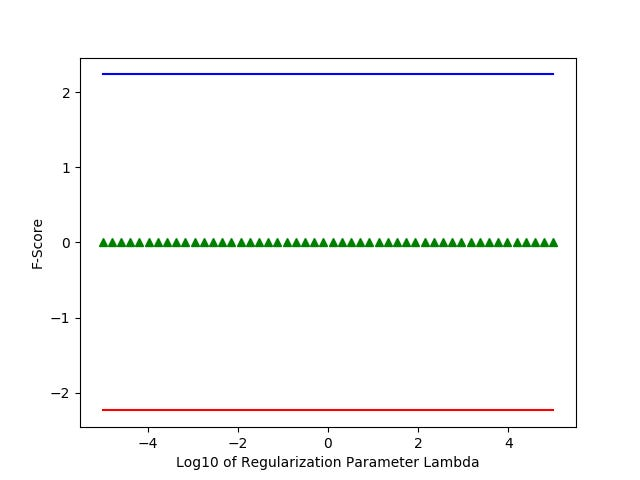Left: “Figure 3 (a): Prior” & Right: “Figure 3 (b): Prior (different perspective)”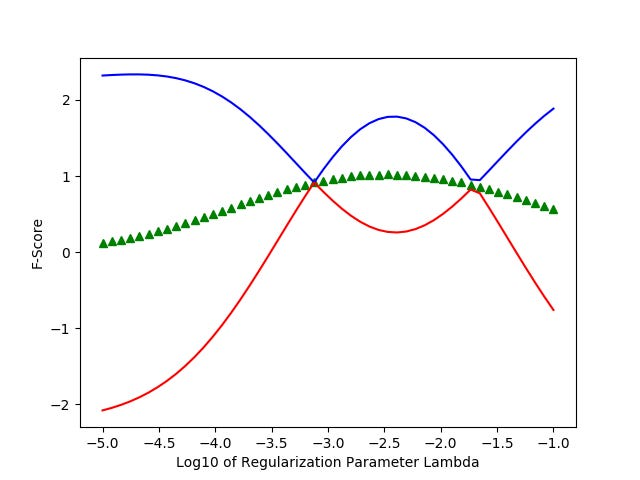Left: “Figure 3 (c): Evaluation 1” & Right: “Figure 3 (d): Evaluation 2”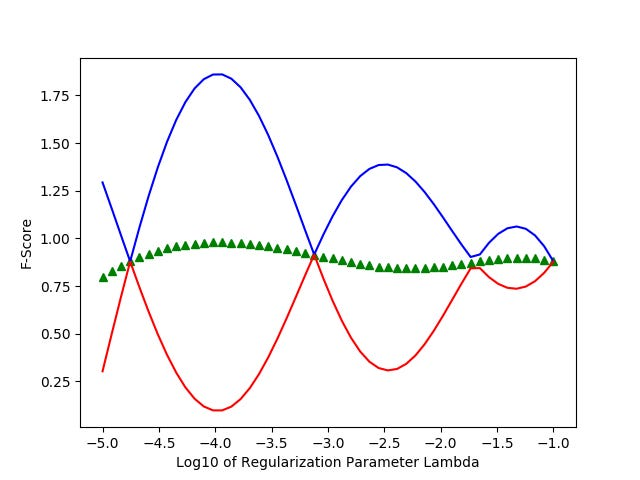Left: “Figure 3 (e): Evaluation 3” & Right: “Figure 3 (f): Evaluation 4”

One downside of Bayesian optimization using Gaussian processes is that it suffers from the curse of dimensionality. This is because we have to discretize the hyperparameter space. However, for many machine learning applications, the hyperparameter space is inherently low dimensional. So this is not a problem in most cases, but just wanted to make the reader aware of the method’s limitations.

As an aside, we could theoretically use Bayesian optimization to update the weights of the neural network without having to compute its gradients and be able to find the global optimum much faster. However, because there are millions of weights involved, the curse of dimensionality (as alluded earlier) makes it computationally infeasible. Hence, it is not used to update the weights of the neural network.

For those familiar with Reinforcement Learning, the above mentioned Bayesian optimization algorithm using Gaussian processes is basically similar to the multi-armed bandit problem studied in Reinforcement Learning, where each discretized hyperparameter is a bandit arm and adjacent bandit arms are strongly correlated. Moreover, the UCB algorithms helps balance the exploration-exploitation tradeoff.

RESULTS
After obtaining the optimal regularization parameter, the network was further trained for 40 additional epochs. The final F-score obtained (between vehicle and road classes) was 0.95 for the validation set and 0.86 for the test set. Figure 4 shows a few examples of the resulting performance on the test images. Note that the vehicle category is highlighted in blue, road is highlighted in green, and the everything else category is not highlighted (i.e same as in the original image). Furthermore, before doing inference, the network weights are frozen and optimized to remove any variables used during training (like gradients etc) that are not needed during inference.

CONCLUSION
A VGG16 based FCN-8 network was trained for the Lyft-Udacity perception challenge and a test set F-score of 0.86 was achieved. Moreover, finding the optimal regularization parameter was performed using Bayesian optimization. The network was trained on an Nvidia K80 GPU on Google cloud, and the inference speed was about 6 FPS, and thus more improvement on the speed and accuracy front is a future goal. Furthermore, instead of sampling for the next hyperparameter using UCB , Thompson sampling can also be used to more quickly find the optimal hyperparameter.

Another thing that can be addressed in the future is to perform Bayesian optimization on all the hyperparameters — i.e. regularization parameter, learning rate, and batch size. For this project, the regularization parameter was optimized using Bayesian optimization because the learning rate and batch size were easier to fine tune by doing random search. While we can run three independent Bayesian optimization algorithms (one for each hyperparameter), it assumes no correlation between the hyperparameters when finding the optimal hyperparameter set, which is not a good assumption. Thus a better way is to perform Bayesian optimization on the three hyperparameters at once (i.e. while taking into account their correlations). Thus another future goal is to do Bayesian optimization using multivariate Gaussian processes.

Lastly, I would like to thank Udacity and Lyft for allowing me to participate in the challege.

REFERENCES
 https://people.eecs.berkeley.edu/~jonlong/long_shelhamer_fcn.pdf
 Nando de Freitas’ CS540 Bayesian optimization Lectures (http://www.cs.ubc.ca/~nando/540-2013/lectures.html)
 Nando de Freitas’ CS540 Gaussian processes Lectures (http://www.cs.ubc.ca/~nando/540-2013/lectures.html)

Source: Deep Learning on Medium